# If-Else Statements in C#

## If-Else Statements in C# with Examples

In this article, I am going to discuss If-Else Statements in C# with Examples. Please read our previous article, where we discussed Control Flow Statements in C# with Examples. The If-Else Statements belong to the Selection Statements or Branching Statements Category. So, before understanding if-else statements, let us first understand what are Selection or Branching Statements in C# Language.

##### What are Selection or Branching Control Flow Statements in C#?

It is also called as Decision Making Statements. Decision Making in a programming language is very much similar to decision-making in real life. For example, you might have one situation where you will decide whether you to go to the office or you want to go theatre to watch a movie. And the condition is that, if it is raining, I will go to the theatre and if it is not raining then I will go to the office. So, the condition is rain, and based on the rain, you will decide what you need to do.

In programming also, we will face some situations where we want a certain block of code to be executed when some condition is fulfilled. The decision-making statements in C# allow us to make a decision, based upon the result of a condition. If the condition is satisfying, we may need to execute some piece of code and if the condition is failed, we might need to execute some other piece of code.

The Selection or Branching or Decision-Making Statements in C# allows us to control the flow of program execution based on some condition. It executes different sections of code depending on a specific condition. Selection statements can be divided into the following categories:

2. Switch Statements (I will discuss in the next article)
##### If Statement in C# Language:

It executes a block of statements (one or more instructions) when the condition in the if block is true and when the condition is false, it will skip the execution of the if block. Using else block is optional in C#. Following is the syntax to use the if block in the C# language.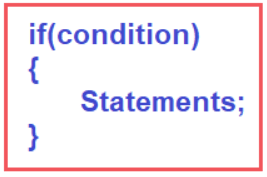##### Flow Chart of If Block:

Let us understand how we will represent the execution flow of the if block using a flow chart. The starting point is represented by the oval symbol. And the flow will be from the condition and the condition is represented by a diamond shape. Here, first, we need to check the condition. And for every condition, two options are there i.e. if conditions are successful (condition is true) and if conditions are failed (condition is false). That means two situations are there i.e. TRUE and FALSE.

Suppose, the condition is TRUE, then all statements are defined inside the if block will get executed. And the statements we are representing in the flow chart with the help of a parallelogram symbol. And after the execution of the statements, the control will come to end. Suppose, the condition is false, then without executing any statement inside the if block, the control will come to the end. For better understanding, please have a look at the below diagram which represents the flow chart of the if conditional statement.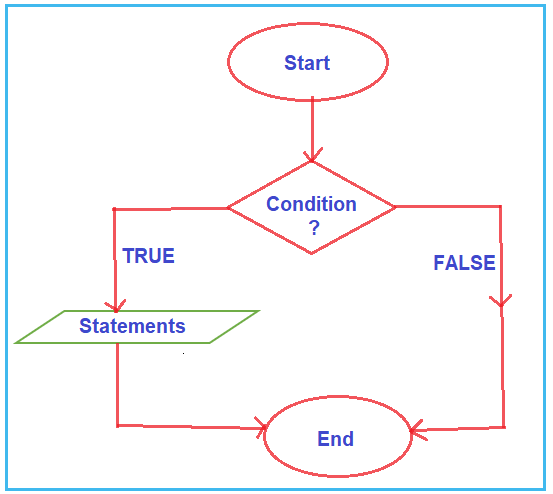Note: Here, the block of statements executes only when the condition is true. And if the condition is false, then it will skip the execution of the statements.

##### Example to Understand If Block in C#:

Let us write a program to check whether the number is greater than 10 using the if statement in C# Language. Here, we will take the number from the user and then we will check whether that number is greater than 10 or not using the if Statement in C# Language.

The following program exactly does the same. In the below program, inside the main method, we are declaring one integer variable i.e. number, and then we are taking the input from the user and storing it in the number variable. After reading the input we are checking whether the given number is greater than 10 or not. If the number > 10, then the condition is true, and, in that case, the two statements that are present inside the if block will be executed else if the condition is false, then the if block statements will be skipped.

```using System;
namespace ControlFlowDemo
{
class Program
{
static void Main(string[] args)
{
int number;
Console.WriteLine("Enter a Number: ");
if (number > 10)
{
Console.WriteLine(\$"{number} is greater than 10 ");
Console.WriteLine("End of if block");
}
Console.WriteLine("End of Main Method");
}
}
}
```
###### Output:

If we take 15 as an input, 15 > 10 means the condition is true, then if block statement gets executed.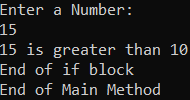If we take 5 as an input, 5 > 10 means the condition is false, then the if block statements will be skipped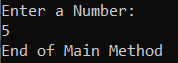##### If Statement without Curly Braces in C# Language

In the declaration of if block if we do not specify statements using blocks {} (curly braces), then only the first statement will be considered as the if block statement. To understand this, please have a look at the below example.

```using System;
namespace ControlFlowDemo
{
class Program
{
static void Main(string[] args)
{
int number;
Console.WriteLine("Enter a Number: ");
if (number > 10)
Console.WriteLine(\$"{number} is greater than 10 ");
Console.WriteLine("End of Main Method");
}
}
}
```

As you can see, in the above example, we have not specified the curly braces to define the if block. In this case, only the first statement which appeared after the if block will be considered as the if block statement. The second statement will not be a part of the if block. For a better understanding, please have a look at the below image. The statement which is in red color will belong to the if block and the statement which is in the black color do not belong to the if block.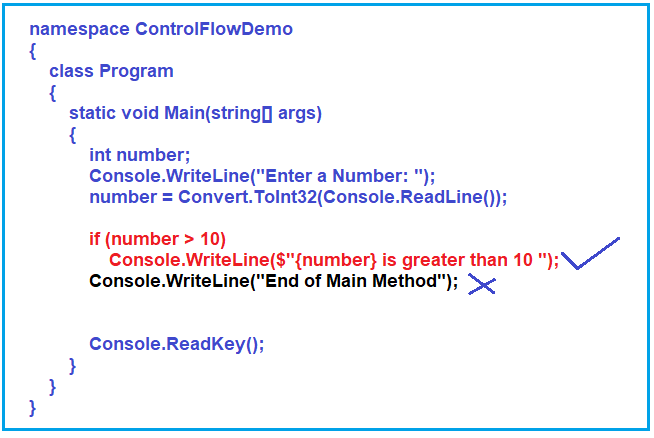So, when you execute the above program, irrespective of the condition, the black statement is always going to be executed as it is not part of the if block. The red statement is only executed when the if condition is true. For a better understanding, please have a look at the below image.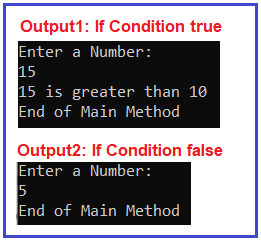Note: The point that you need to keep in mind is that if you have a single statement for if block, then you can represent that statement either using curly braces or without using curly braces. But if you have more than one statement inside the if block, then it is mandatory to use curly braces. I like to use curly braces in my programming even if the if condition contains a single statement. The curly braces explicitly specify where the if block started and where it ended.

##### If Else Statements in C# Language:

The If-Else block in C# Language is used to provide some optional information whenever the given condition is FALSE in the if block. That means if the condition is true, then the if block statements will be executed, and if the condition is false, then the else block statement will execute.

So, in simple words, we can say that, if we want to execute some statement(s) when the condition is true and we also want to execute some other statement(s) when the condition is false, then, in that case, we need to use IF-ELSE conditional statements in C#. Following is the syntax to use the IF ELSE block in the C# language.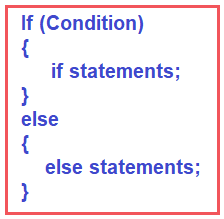Note: The point that you need to remember is that only one block of statement i.e. either if block or else block is going to be executed at a time. So, if the condition is TRUE if block statements get executed and if the condition is FALSE, then else block statements get executed.

##### Is it mandatory to use else block?

No, it is not mandatory to use else block. It is an optional block. You can use only if block also. If you want to provide some information when the condition failed, then you need to use this optional else block.

##### Flow Chart of If-Else Block:

The flow chart of the if-else block is almost similar to the if block. In this case, when the condition is true, the if block statements get executed and when the condition is false, the else block statements get executed. For better understanding, please have a look at the below image which shows the flow chart of the if-else block.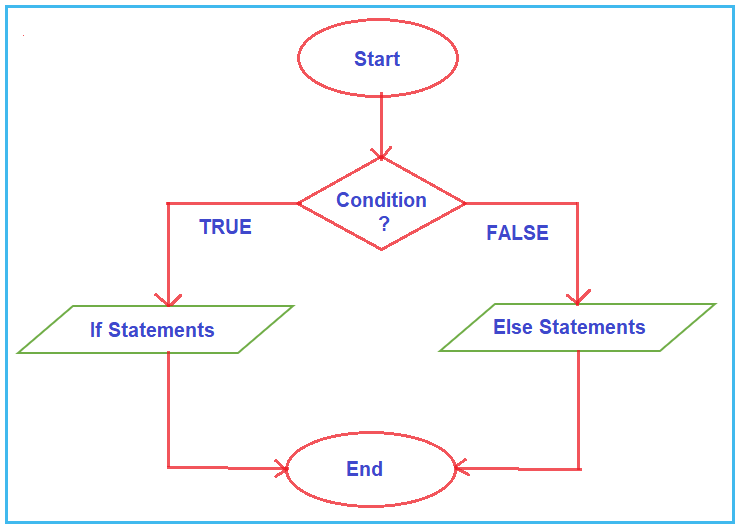Note: In C# Programming Language, if and else are reserved words. That means you cannot use these two keywords for the naming of any variables, properties, class, methods, etc. The expressions or conditions specified in the if block can be a Relational or Boolean expression or condition that evaluates to TRUE or FALSE. Now let us see some examples to understand the if-else conditional statements.

##### Example to Understand IF-ELSE Statement in C#:

Let us write a Program to Check Whether a Number is Even or Odd using If Else Statements in C# Language. Here we will take the input number from the user and then we will check whether that number is even or odd using the if-else statement in C# Language. The following program exactly does the same.

```using System;
namespace ControlFlowDemo
{
class Program
{
static void Main(string[] args)
{
Console.WriteLine("Enter a Number: ");
if (number % 2 == 0)
{
Console.WriteLine(\$"{number} is an Even Number");
}
else
{
Console.WriteLine(\$"{number} is an Odd Number");
}

}
}
}```

In the above program, inside the main method, we are declaring one integer variable i.e. number and then we are reading input from the user and storing the value in the number variable. After reading the input we are checking whether the given number is even or odd. An Even number is a number that is divisible by 2. If number % 2 equals 0, then the if condition is true and, in that case, we are printing a message that it is an even number and if the condition is false then we are printing a message that it is an odd number.

For example,

If we take 16 as an input, 16%2 == 0 means the condition is true, then the if block statement gets executed. And the output will be 16 is an Even Number.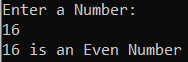If we take 13 as an input, 13%2 == 0 means the condition is false, then the else block statements get executed. And the output will be 13 is an Odd Number.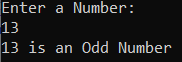##### If and Else Block without Curly Braces in C# Programming Language

In the declaration of if block or else block if we do not specify statements using curly braces {}, then only the first statement will be considered as the if block or else block statement. Let us understand this with an example. Please have a look at the below code.

```using System;
namespace ControlFlowDemo
{
class Program
{
static void Main(string[] args)
{
int number = 10;
if (number == 10)
Console.WriteLine("Hi"); //This Statement belongs to IF Block
else
Console.WriteLine("Hello"); //This Statement belongs to ELSE Block
Console.WriteLine("Bye");

}
}
}```

As you can see, in the above example, while creating the if and else block we have not specified the curly braces. So, in this case, the first Console.WriteLine statement will belong to the if block. After the else statement, we have two Console.WriteLine statements. Here, the Console.WriteLine statement which printing the Hello message will belongs to the else block only. The next Console.WriteLine statement which printing the message bye will not belong to else block. Now, if you execute the above program then you will get the following output.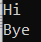Now, if we replace the Hello statement inside the if block, then we will get an ERROR. The reason is that not more than one statement gets executed without braces. One statement will execute inside the if block. For better understanding, please have a look at the below example.

```using System;
namespace ControlFlowDemo
{
class Program
{
static void Main(string[] args)
{
int number = 10;
if (number == 10)
Console.WriteLine("Hi");
Console.WriteLine("Hello");
else
Console.WriteLine("Bye");

}
}
}
```

If we want to execute more than one statement then you should use braces and all the statements will be inside the braces. The following code works fine. Here, we are using curly braces in the if block.

```using System;
namespace ControlFlowDemo
{
class Program
{
static void Main(string[] args)
{
int number = 10;
if (number == 10)
{
Console.WriteLine("Hi");
Console.WriteLine("Hello");
}
else
Console.WriteLine("Bye");

}
}
}
```

Note: For every if condition statement, the else block is optional. But for every else block, the if block is compulsory. The purpose of the ‘if’ statement in a program is to allow multiple execution paths for varying user inputs, making it more interactive!

##### Nested If-Else Statements in C# Language:

When an if-else statement is present inside the body of another if or else then this is called nested if-else. Nested IF-ELSE statements are used when we want to check for a condition only when the previous dependent condition is true or false.

##### What is the Nested If block?

Nested if block means defining if block inside another if block. We can also define the if block inside the else blocks. Depending on our logic requirements, we can use nested if block either inside the if block or inside the else. Please have a look at the below image which shows the different ways to use the nested if block in C# Language.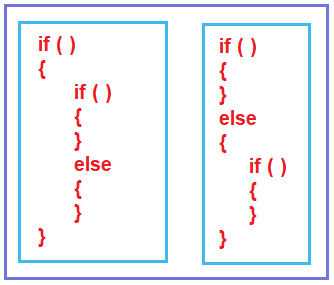Now, we will take one example and try to understand the flow chart. We are taking the following syntax. Here, we have an if-else block inside the if block, as well as, an if-else block inside the else block.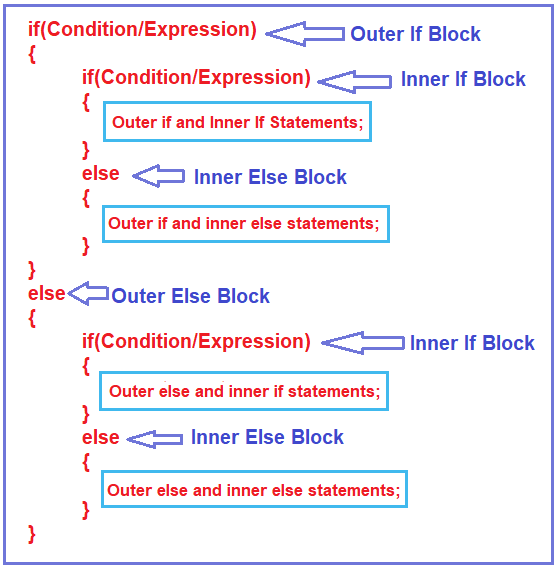Let us understand the above code.

1. Condition1: First, it will check the first if condition i.e. the outer if condition and if it is true, then the outer else block will be terminated. So, the control moves inside the first or the outer if block. Then again it checks the inner if condition and if the inner if condition is true, then the inner else block gets terminated. So, in this case, the outer if and inner if block statements get executed.
2. Condition2: Now, if the outer if condition is true, but the inner if condition is false, then the inner if block gets terminated. So, in this case, the outer if and inner else block statements get executed.
3. Condition3: Now, if the outer if condition is false, then the outer if block gets terminated and control moves to the outer else block. And inside the outer else block, again it checks the inner if condition, and if the inner if condition is true, then the inner else block gets terminated. So, in this case, the outer else and inner if block statements get executed.
4. Condition4: Now, if the outer if condition is false as well as the if condition inside the outer else blocks also failed, then the if block gets terminated. And in this case, the outer else and inner else block statements get executed. This is how statements get executed in Nested if. Now we will see the flow chart of nested if blocks.
##### Flow Chart of Nested If – Else Block in C# Language:

Please have a look at the below diagram which shows the flow chart of the nested if-else statement.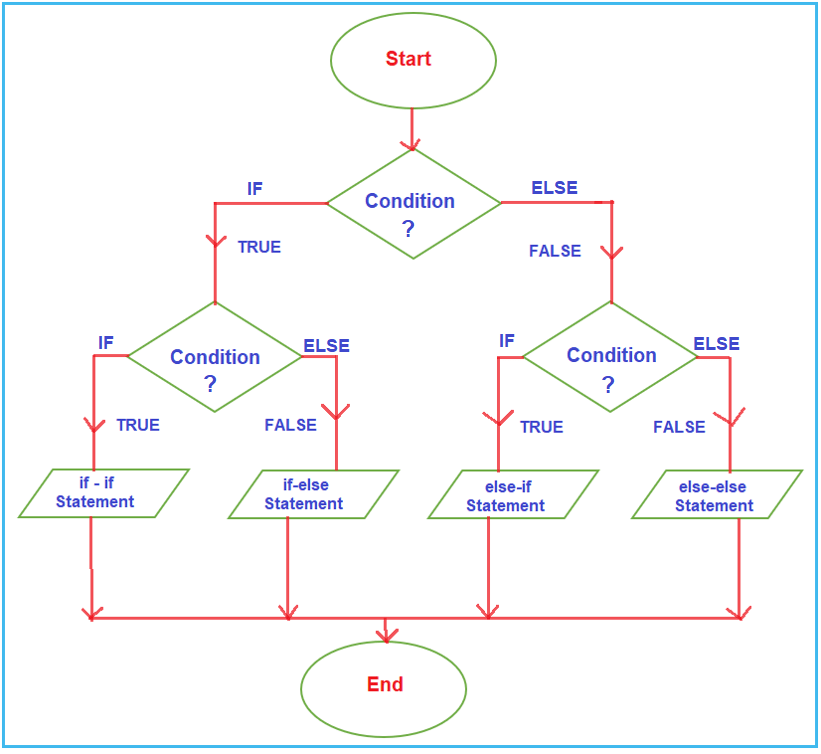First, it will check the outer if condition, and if the outer if condition is true, then the control comes inside and it will check the inner if condition, and if the inner if condition is true, then the outer if block statements and inner if block statements get executed. And after execution, it will come to end.

Suppose, the outer if condition is true but the inner if condition is failed, then the outer if block statements and the inner else block statement get executed. And once the statement gets executed, it will come to end.

Suppose, the outer if condition failed, then the control directly comes to the else block and checks the inner if condition. And again, for the inner if condition two options are there. If the inner if condition is true, then it will execute the outer else block and inner if block statement, and if the inner if condition is false, then it will execute the outer else block and inner else block statements and then comes to end.

##### Example to understand Nested IF-ELSE Statements in C# Language:

In the below example, we are finding the largest number between three numbers using nested IF-ELSE statements.

```using System;
namespace ControlFlowDemo
{
class Program
{
static void Main(string[] args)
{
int a = 15, b = 25, c = 10;
int LargestNumber = 0;

if (a > b)
{
Console.WriteLine(\$"Outer IF Block");
if (a > c)
{
Console.WriteLine(\$"Outer IF - Inner IF Block");
LargestNumber = a;
}
else
{
Console.WriteLine(\$"Outer IF - Inner ELSE Block");
LargestNumber = c;
}
}
else
{
Console.WriteLine(\$"Outer ELSE Block");
if (b > c)
{
Console.WriteLine(\$"Outer ELSE - Inner IF Block");
LargestNumber = b;
}
else
{
Console.WriteLine(\$"Outer ELSE - Inner ELSE Block");
LargestNumber = c;
}
}

Console.WriteLine(\$"The Largest Number is: {LargestNumber}");

}
}
}
```
###### Output: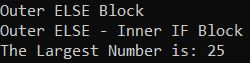As in our previous article, we have discussed, sometimes it is possible to convert the if-else statement to a ternary condition. Let us rewrite the above example using the ternary operator to find the biggest number between the three numbers.

```using System;
namespace ControlFlowDemo
{
class Program
{
static void Main(string[] args)
{
int a = 15, b = 25, c = 10;
int LargestNumber = 0;

Console.WriteLine(\$"a={a}, b={b}, c={c}");
LargestNumber = (a > b) ? ((a > c)?(a):(c)) : ((b > c) ?(b):(c));

Console.WriteLine(\$"The Largest Number is: {LargestNumber}");

}
}
}
```
###### Output: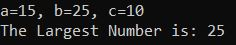##### Ladder if-else statements in C# Language:

In Ladder if-else statements one of the statements will be executed depending upon the truth or false of the conditions. If the condition1 is true then Statement 1 will be executed, and if condition2 is true then statement 2 will be executed, and so on. But if all conditions are false, then the last statement i.e. else block statement will be executed. The C# if-else statements are executed from top to bottom. As soon as one of the conditions controlling the if is true, the statement associated with that if block is going to be executed, and the rest of the C# else-if ladder is bypassed. If none of the conditions are true, then the final else statement will be executed.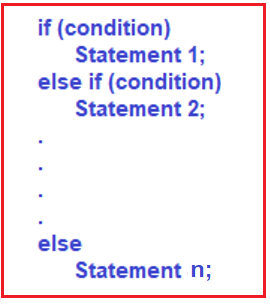##### Example to understand Ladder If-Else Statements in C# Language:
```using System;
namespace ControlFlowDemo
{
class Program
{
static void Main(string[] args)
{
int i = 20;
if (i == 10)
{
Console.WriteLine("i is 10");
}
else if (i == 15)
{
Console.WriteLine("i is 15");
}
else if (i == 20)
{
Console.WriteLine("i is 20");
}
else
{
Console.WriteLine("i is not present");
}

}
}
}
```

Output: i is 20

1.